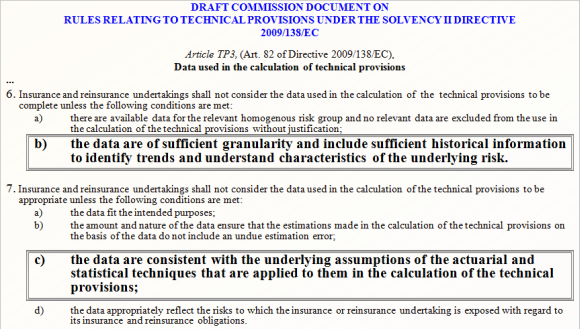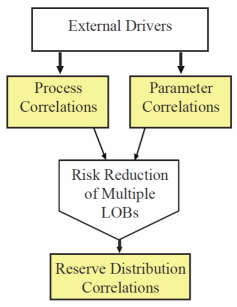# Critical Information required for Solvency II calculations

In order to compute Technical Provisions (TP), Market Value Margins (MVMs) using the Cost of Capital approach and Solvency Capital Requirement (SCR) for the one-year risk horizon for the aggregate of all long tail LOBs and each LOB, the following critical information is required:• Probability distributions of the paid losses (liability stream) by calendar year (k=1,...,n), and their correlations for each LOB and the aggregate of all LOBs where complete run-off is achieved at the ultimate calendar year n.
• Probability distributions of the above conditional on the first calendar year's losses being at the 99.5th percentile, i.e. the year is "in distress" (denoted ξ).

Armed with the above distributions, any risk measure can be computed, including VaR(k) and VaR(k|ξ) for calendar year k, for each LOB, and the aggregate of all LOBs.

Correlations between calendar year liability streams are a function of parameter uncertainty and process variability.

Between LOBs there are three types of correlations: process correlation, parameter correlation and reserve distribution correlation.## Correlations between multiple LOBs

Process correlation is the correlation in the pure volatility component of the liabilities. This is measured after all trends have been accounted for. Parameter correlation can arise independently due to common external effects, but is also induced via process correlation, since the estimation of model parameters depends on data subject to correlated random effects.

Modelling multiple LOBs leads to risk diversification credit that is very highly dependent on the correlations between the LOBs.

For example: Lines A and B have a process correlation of 0.5, but a reserve distribution correlation of 0.1. Individually the 99.5% VaRs are \$596M and \$602M. If they were treated as uncorrelated the 99.5% VaR of the aggregate is \$800M, but if the correlation (0.1) is correctly factored in this figure increases to \$842M. In this case an accurate estimation of reserve distribution correlation leads to a 5% increase in risk funds over the assumption of full diversification. The cost of ignoring the correlation is greater however, as an unplanned increase in required solvency funds can negatively impact a company's future financial position.

• Solvency II

(c) Insureware 1985 ~ 2022

Insureware Pty. Ltd.
info@insureware.com